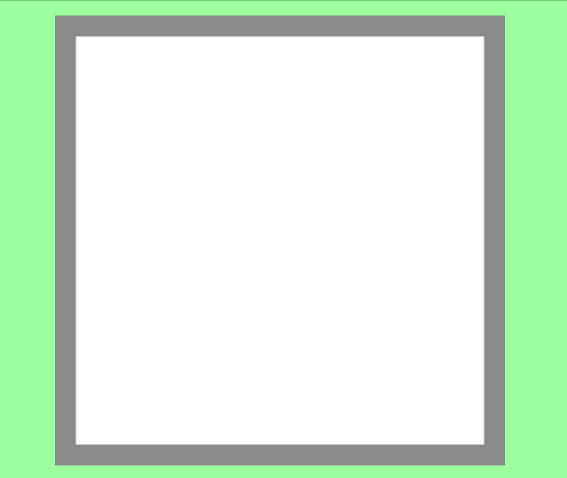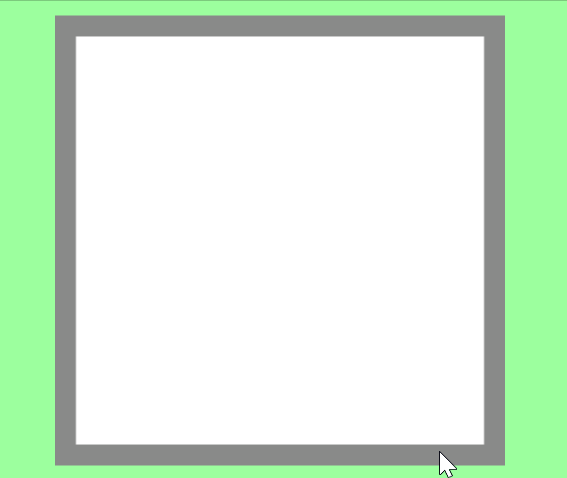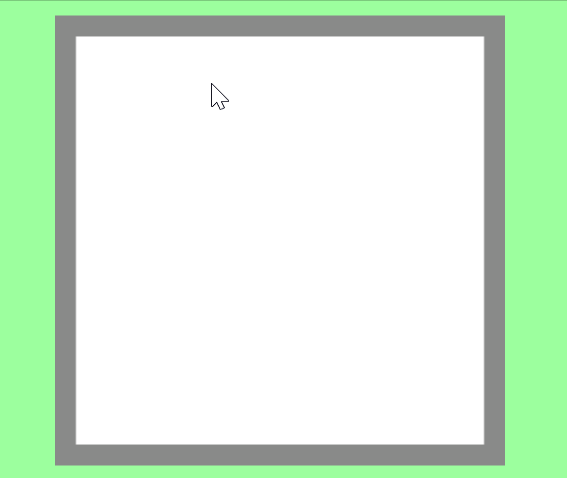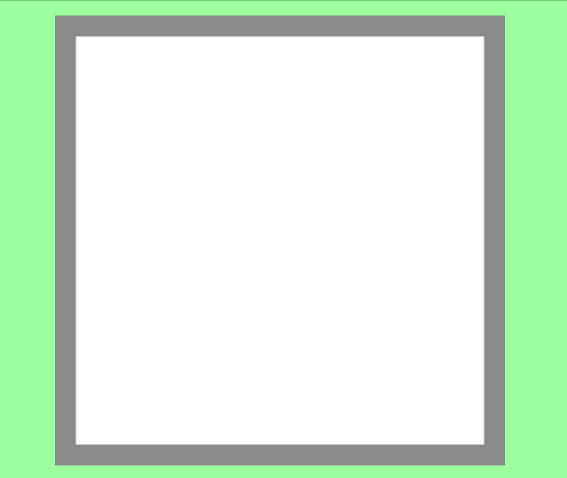# [Unity (C #)] Draw smooth lines with Lerp

## Introduction

I investigated how to draw a line smoothly on a plane.

I referred to an example of slimy movement even in VR space (↓ amazing)

## Implementation to draw

I borrowed the code of “drawing part” from the link below.

## demo

First of all, it is a sample that I tried to move as it is without interpolation.The line is faint and it is awkward.

Next, it is interpolated by Lerp.He firmly interpolated between the lines.

## code

The full text below.

``````using UnityEngine;

//How to use ↓
//https://nn-hokuson.hatenablog.com/entry/2016/12/08/200133
public class SmoothPaint : MonoBehaviour
{
Texture2D drawTexture;
Color[] buffer;

private Vector2 _prevPosition;

void Start()
{
Texture2D mainTexture = (Texture2D) GetComponent<Renderer>().material.mainTexture;
Color[] pixels = mainTexture.GetPixels();

buffer = new Color[pixels.Length];
pixels.CopyTo(buffer, 0);

drawTexture = new Texture2D(mainTexture.width, mainTexture.height, TextureFormat.RGBA32, false);
drawTexture.filterMode = FilterMode.Point;
}

public void Draw(Vector2 p)
{
for (int x = 0; x < 256; x++)
{
for (int y = 0; y < 256; y++)
{
if ((p - new Vector2(x, y)).magnitude < 5)
{
buffer.SetValue(Color.black, x + 256 * y);
}
}
}
}

void Update()
{
if (Input.GetMouseButton(0))
{
//If the previous value does not exist yet, treat the current value as the previous value
if (_prevPosition == Vector2.zero)
{
_prevPosition = Input.mousePosition;
}

//Input endpoint coordinates used for linear interpolation
Vector2 endPosition = Input.mousePosition;
//1 frame line distance
float lineLength = Vector2.Distance(_prevPosition, endPosition);
//Interpolated value that changes according to the length of the line CeilToInt rounds up after the decimal point
int lerpCount = Mathf.CeilToInt(lineLength / lerpCountAdjustNum);

for (int i = 1; i <= lerpCount; i++)
{
//Lerp percentage value"Current number of times/Total number of times"Put out with
float lerpWeight = (float) i / lerpCount;

//Calculate the coordinates to be interpolated by passing the previous input coordinates, the current input coordinates, and the ratio.
Vector3 lerpPosition = Vector2.Lerp(_prevPosition, Input.mousePosition, lerpWeight);

Ray ray = Camera.main.ScreenPointToRay(lerpPosition);
RaycastHit hit;
if (Physics.Raycast(ray, out hit, 100.0f))
{
Draw(hit.textureCoord * 256);
}

drawTexture.SetPixels(buffer);
drawTexture.Apply();
GetComponent<Renderer>().material.mainTexture = drawTexture;
}

//Record the previous input coordinates
_prevPosition = Input.mousePosition;
}
else
{
//Reset the previous input coordinates
_prevPosition = Vector2.zero;
}
}
}
``````

The logic is as follows
** ① Hold the mouse input coordinates of the previous frame
② Calculate the distance between ① and the current input coordinates in the next frame
③ Calculate the interpolation value (number of interpolations) according to the distance
④ Interpolate with Lerp using the interpolated value **

## Lines connect

I implemented it and spent a fair amount of time
It was a phenomenon that ** lines were connected **.

With the newly started drawing like the GIF below
The last end point was connected.This keeps the value at the end of drawing as the previous value
The cause was that we were interpolating at the new input location.

Therefore, when there is no input ** in the following places, the previous value is reset **.
In addition, due to the reset of the input value, the frame where the previous value does not exist,
That is, ** there is no need to perform interpolation in the frame at the beginning of drawing **, so
The previous value = the current value.

``````
if(Input.GetMouseButton(0))
{
//If the previous value does not exist yet, treat the current value as the previous value
if (_prevPosition == Vector2.zero)
{
_prevPosition = Input.mousePosition;
}
}
else
{
//Reset the previous input coordinates
_prevPosition = Vector2.zero;
}
``````

This allowed me to draw a new line where I started drawing.## in conclusion

If you calculate the interpolation value considering the line thickness (size)
It seems that more optimal interpolation can be done.

If I can write it, I will write it.

Tags:

Updated: